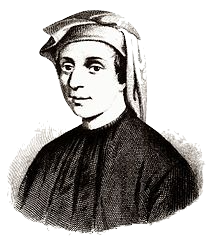Blog of Andrés Aravena
CMB2:

# Homework 4

08 March 2019. Deadline: Friday, 15 March, 9:00.

# 1. RabbitsFigure 1. Leonardo Fibonacci, expert on rabbits and numbers.

Near 1170, in the Italian city of Pisa, the son of Guglielmo Bonacci was born. He was named Leonardo. Nowadays, he is known as Leonardo Pisano (meaning “Leonardo of Pisa”) and also as Leonardo Fibonacci (short for “filius Bonacci”, that is, “son of Bonacci”).

Leonardo traveled with his father and, while staying in the city of Béjaïa (Algeria), he was sent to study calculation with an Arab master. He later went to Egypt, Syria, Greece, Sicily, and Provence, where he studied different numerical systems and methods of calculation. He soon realized the many advantages of the Hindu-Arabic system, as introduced first by the famous mathematician Muḥammad ibn Mūsā al-Khwārizmī (born c. 780—died c. 850 in Baghdad).

In 1202, Leonardo wrote the “Book of Calculation” which helped europeans to learn the Hindu–Arabic numerals. In that book he wrote:

A certain man put a pair of rabbits in a place surrounded on all sides by a wall. How many pairs of rabbits can be produced from that pair in a year if it is supposed that every month each pair begets a new pair which from the second month on becomes productive?

• The population begins in the first month with a pair of newborn rabbits.
• Rabbits reach reproductive age after one month.
• In any given month, every rabbit of reproductive age mates with another rabbit of reproductive age.
• Exactly one month after two rabbits mate, they produce one male and one female rabbit.
• Rabbits never die or stop reproducing.

Fibonacci’s exercise was to calculate how many pairs of rabbits would remain in one year. We can see that in the second month, the first pair of rabbits reach reproductive age and mate. In the third month, another pair of rabbits is born, and we have two rabbit pairs; our first pair of rabbits mates again. In the fourth month, another pair of rabbits is born to the original pair, while the second pair reach maturity and mate (with three total pairs). After a year, the rabbit population has 144 pairs.

Beyond rabbits, it has been observed that Fibonacci numbers appear often in nature; for example, in the spirals of sunflower heads, in pine cones, in the genealogy of the male bee, in the spiral in snail shells, in the arrangement of leaf buds on a stem, and in animal horns. You can see some of them looking for “Fibonacci in nature” on Google images.

Doing some abstraction, we can use fib(n) to represent the number of pairs of rabbits on month n. The numbers have the following rules:

• fib(0) = 0
• fib(1) = 1
• fib(n) = fib(n-1) + fib(n-2)

Your task is to write a recursive function in R that has input n and output fib(n). Remember that recursive means that the function calls itself, like in the factorial example. You can test your function by checking that at the end of the first year there are 144 pairs of rabbits.

# 2. Finding the other DNA strand

FASTA files contain only one of the two strands of DNA molecules. The second strand can be calculated by reversing and complementing the first strand. Reversing means that the second strand is created from the last to the first nucleotide. Complement means that each nucleotide of the first strand is in front of its Watson-Crick pair.

For example, the reverse-complement of ATGACATAGTG is CACTATGTCAT.

Please write the code for the following function:

reverse_complement <- function(dna) {
# create reverse complement
}

# 3. Calculating GC skew to find replication origin

We want to find the Origin of Replication of E.coli. To find it, we need to see how the GC skew changes through the genome. In other words, we need a function to compute the GC skew in different positions of the genome.

Write code for this function

gc_skew <- function(dna, position, window_size) {
# code here
return(skew)
}

Then use a for(){} loop to evaluate this function in non-overlapping windows of size 1000bp. Draw the resulting vector.

# Delivery

Please send me the homework4.R file in a single email to andres.aravena+cmb@istanbul.edu.tr. Do not forget to write your name and number.